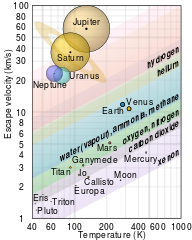Cococubed.com Thermal Escape

Home

Astronomy research
Software instruments
Stellar equation of states
EOS with ionization
EOS for supernovae
Chemical potentials
Stellar atmospheres

Voigt Function
Jeans escape
Polytropic stars
Cold white dwarfs

Cold neutron stars
Stellar opacities
Neutrino energy loss rates
Ephemeris routines
Fermi-Dirac functions

Polyhedra volume
Plane - cube intersection
Coating an ellipsoid

Nuclear reaction networks
Nuclear statistical equilibrium
Laminar deflagrations
CJ detonations
ZND detonations

Fitting to conic sections
Unusual linear algebra
Derivatives on uneven grids

Supernova light curves
Exact Riemann solutions
1D PPM hydrodynamics
Hydrodynamic test cases
Galactic chemical evolution

Universal two-body problem
Circular and elliptical 3 body
The pendulum
Phyllotaxis

MESA
MESA-Web
FLASH

Zingale's software
Brown's dStar
GR1D code
Herwig's NuGRID
Meyer's NetNuc
Presentations
Illustrations
Public Outreach
Education materials
2023 ASU Solar Systems Astronomy
2023 ASU Energy in Everyday Life

AAS Journals
2023 AAS Peer Review Workshops
2023 MESA VI
2023 MESA Marketplace
2023 MESA Classroom
2023 Neutrino Emission from Stars
2023 White Dwarfs & 12C(α,γ)16O
2022 Earendel, A Highly Magnified Star
2022 Black Hole Mass Spectrum
2022 MESA in Don't Look Up
2021 Bill Paxton, Tinsley Prize

Contact: F.X.Timmes
my one page vitae,
full vitae,
research statement, and
teaching statement.

Given a planet's mass ${\rm M}$, radius ${\rm R}$, and the height of its exosphere ${\rm h}$ at temperature ${\rm T}$, the thermal escape timescale $\tau_{\rm escape}$ for an atom/molecule of mass ${\rm m}$ can be estimated from $${\rm \tau_{escape} = \dfrac{H}{v_{jeans}} } \label{eq1} \tag{1}$$ where the scale height ${\rm H}$ and acceleration due to gravity ${\rm g}$ are $${\rm H =\dfrac{k \ T}{m \ g} } \qquad \qquad {\rm g = \dfrac{G \ M} {(R + h)^2} } \label{eq2} \tag{2}$$ and the Jeans speed ${\rm v_{Jeans}}$ is $${\rm v_{jeans} = v_{{\rm peak}} \dfrac{(1+ \lambda) \ e^{-\lambda}}{\sqrt{4 \pi}} } \ . \label{eq3} \tag{3}$$ The peak speed ${\rm v_{peak}}$, escape speed ${\rm v_{escape}}$, and their ratio $\lambda$ are $${\rm v_{peak} = \sqrt{\dfrac{2 k T}{m}} } \qquad \qquad {\rm v_{escape} = \sqrt {\dfrac{2 G M}{R + h}} } \qquad \qquad {\rm \lambda = \left ( \dfrac{ v_{escape}}{v_{peak}} \right )^2 } \label{eq4} \tag{4}$$
The tool contained in public_jeans_escape.tbz implements these simple analytic formulas along with comparing their results to numerical integrations of the Maxwell-Boltzmann distribution. Here are the speed distributions for hydrogen helium, carbon, nitrogen, and oxygen for Earth's exosphere and their associated thermal escape timescales.

Please cite the relevant references if you publish a piece of work that use these codes, pieces of these codes, or modified versions of them. Offer co-authorship as appropriate.